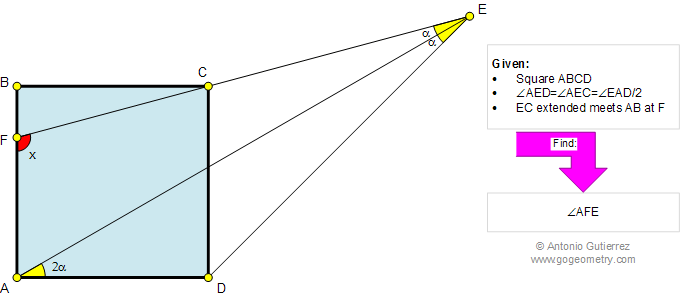Online Geometry Problem 818: Square, Triangle, Angles. Level: High School, SAT Prep, College, Mathematics Education

 The figure below shows a square ABCD with lines EA, ED, and ECF. If angle AED = angle AEC = angle EAD/2, find the measure of angle AFE. Try to use elementary geometry (Euclid's Elements).Home | Search | Geometry | Problems | All Problems | Open Problems | Visual Index | 10 Problems | 811-820 | Triangles | Square | Angles  Email | Solution / comment | By Antonio Gutierrez